Length of conveyors = 10 m

Diameter of driving roller = 90 mm

Diameter of roller bearing=60 mm

Slope of conveyors=5 degree

For drawing model firm use solid Works. Solid Works is solid modelling software that allows you to design products in 3 dimensions. The technique is generally to sketch 2D profiles then use methods like extruding and lofting to produce the solid shape. Solid Works drawings were available as the company had previously designed and manufactured the same feature conveyors. That’s why we did not drawn again. Only, for the conveyors to change the load, motor selection, reducer gear selection, moment analysis, take-off time calculations were made.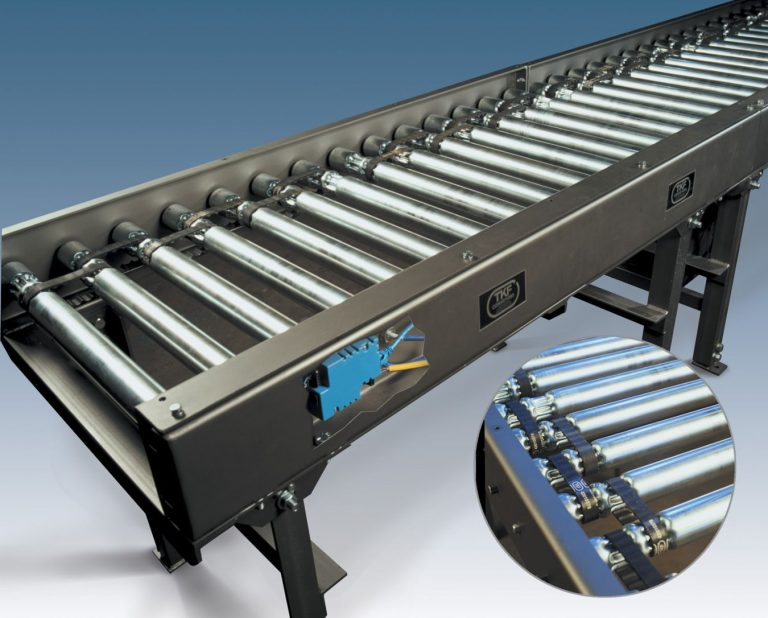#### Conveyor Analysis

The desired mechanical properties for these conveyors are below.

Velocity of conveyors=0,2 m/s

efficiency=0,75 assumption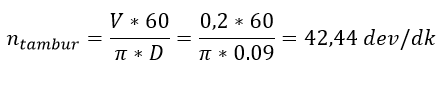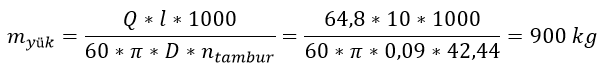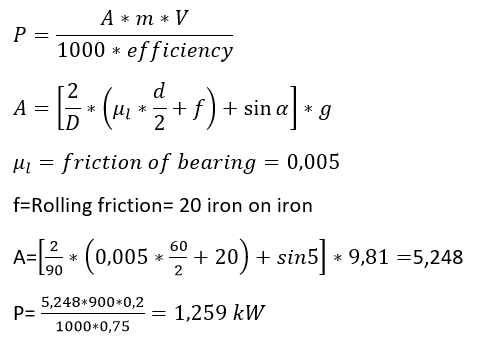#### POWER NEED TO MOVE CONVEYOR

For Conveyor Design, motors and gearboxes in the market close to the desired specifications are given in the table below.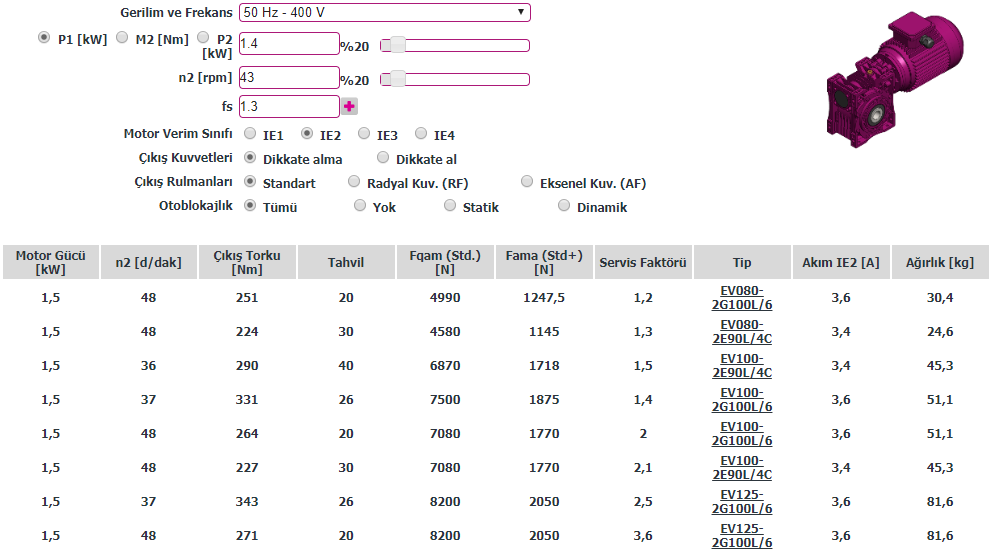From this table the engine power of 1.4 kW, the high revolutions 43 rpm, the lighter and the lighter engine 1 were preferred. Accordingly, moment analysis and service factor were checked. Finally, the take-off time was checked.

Moment Analysis

The table torque values are analyzed according to the serial number of the selected motor.

Tip = EV080-2E100L/6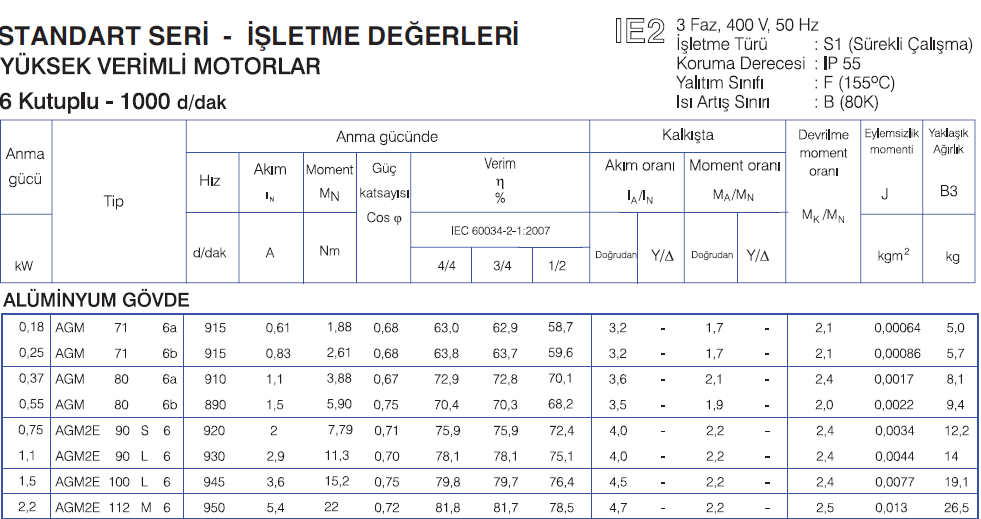From table

Nominal moment of motor =15,2 N.m (Mn)

Moment of inertia =0,0077 kg.m^2

Startup moment ratio =2.2

Startup moment =2.2*1.5=33,14 N.m

J top=J motor+(J reduce/efficiency)=0,0077+=0,01362 kg.m^2

Required moment of inertia to rotate the load

Ml=9550*p/n motor revolution=9550*1,259/860=13,295 N.m

33,14 N.m>13,295 N.m  ok

Motor and Gear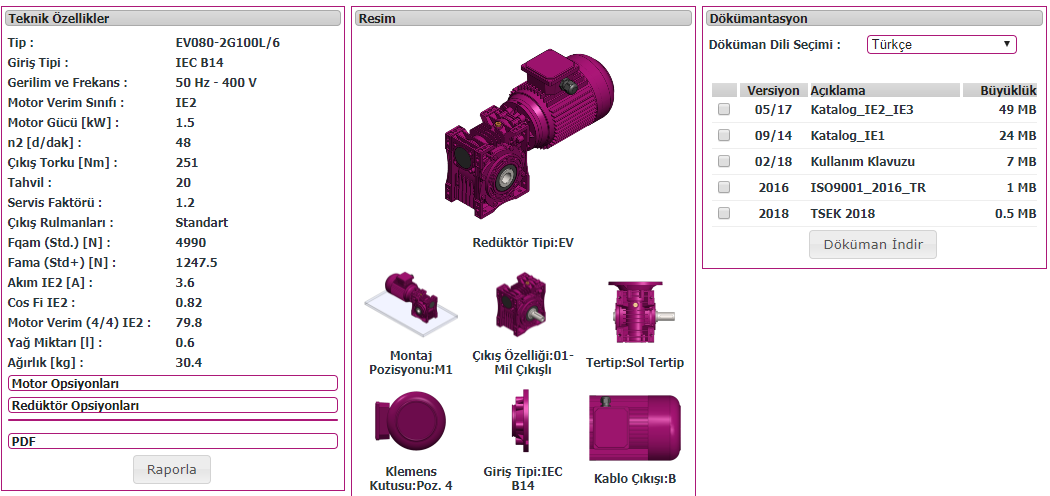50-60 hz & 400 V

P motor power=1.259 kW

n (rpm)=43 rev/min

Tip = EV080-2G100L/6

6 poles =2-4-6-8 poles=3000-1500-1000-750 rpm

L=length of body S-M-L

100 = height of shaft axis =100 mm (56-63-71……..355)

2G=efficient type E2: high efficiency E3:Premium efficiency

EV080 Type of reducer gear

Transformation=20 from table